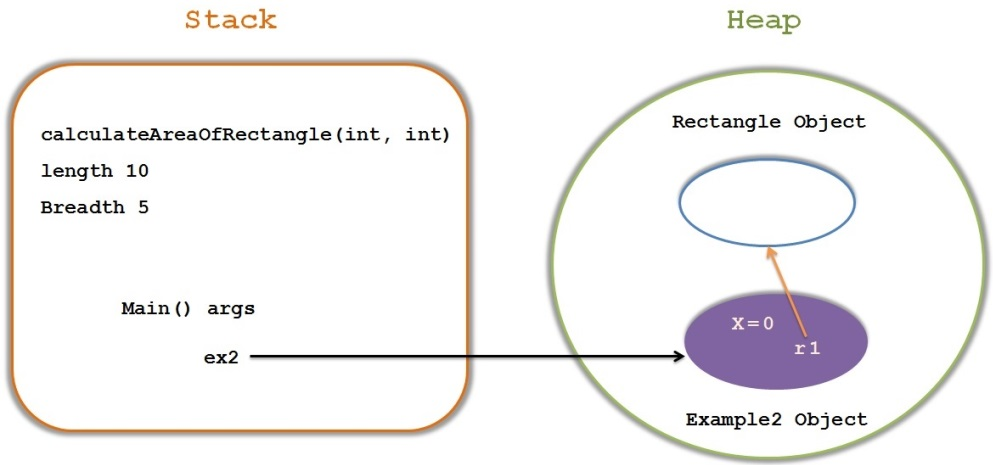# Heap and Stack

`Stack `and `Heap `are the main areas of memory in Java.

##### Before Understanding Stack and Heap , Lets understand below terms in Java.

Local Variables : variables which are declared inside a method or passed as a method parameter.

Instance Variables : Variables which are declared directly inside a class

Example

1. package com.kb.memorymanagement;
2.
3. public class Example1 {
4.
5.     int x;
6.
7.     public void display(int y){
8.         System.out.println(y);
9.     }
10.
11. }
```package com.kb.memorymanagement;

public class Example1 {

int x;

public void display(int y){
System.out.println(y);
}

}```

In the above example, we have one instance variable ‘x’ and one local variable ‘y’.

Now let’s see where these variables reside/live.

All local variables reside inside the `Stack `as long as the method is executing.

Once the method completes the execution, all the variables will be removed from the `stack`.

All instance variables represents the fields of each Object(which can be filled with different value for each instance).

They live inside the object they belong to.

All Objects live inside the `Heap`.

All method invocations reside in `Stack`.

Stack memory size is very less compared to Heap memory.

##### Let’s understand in detail

Example2.java

1. package com.kb.memorymanagement;
2. public class Example2 {
3. float x;
4. Rectangle r1=new Rectangle();
5.
6. public static void main(String[] args) {
7.     Example2 ex2 = new Example2();
8.     ex2.x=ex2.r1.calculateAreaOfRectangle(10, 5);
9. }
10.
11. }
```package com.kb.memorymanagement;
public class Example2 {
float x;
Rectangle r1=new Rectangle();

public static void main(String[] args) {
Example2 ex2 = new Example2();
ex2.x=ex2.r1.calculateAreaOfRectangle(10, 5);
}

}```

Rectangle.java

1. class Rectangle{
2.
3.     public float calculateAreaOfRectangle(int length,int breadth){
4.
6.     }
7. }
```class Rectangle{

}
}```

##### The stack and Heap representation of the above program is as belowFirst Main() method starts the execution and hence this method is loaded into the stack.

`args `and `ex2 `are local variables of Main method, hence they get stored in `Stack`.

Next `calculateAreaOfRectangle()` method getting called by the main method and hence main method stops the execution and `calculateAreaOfRectangle `method gets loaded into the Stack.

length and breadth are local variables of `calculateAreaOfRectangle()` method and hence gets stored on Stack.

Since we have 2 objects in the Heap, one is Example2 object and other one is Rectangle object. both are stored on `Heap`.

Instance variables `x `and `r1 `gets stored inside the Example2 Object inside `Heap`.

##### Remember :

All Local Variables and method calls gets stored in `Stack`, But Instance variables and Objects reside inside the `Heap`.

We can use -Xms and -Xmx JVM option to define the startup size and maximum size of heap memory.

We can use -Xss to define the stack memory size.

When `stack `memory is full, Java runtime throws `java.lang.StackOverFlowError`

whereas if `heap `memory is full, it throws `java.lang.OutOfMemoryError: Java Heap Space error`